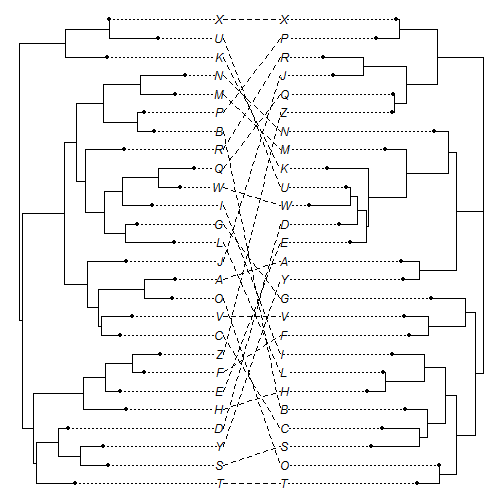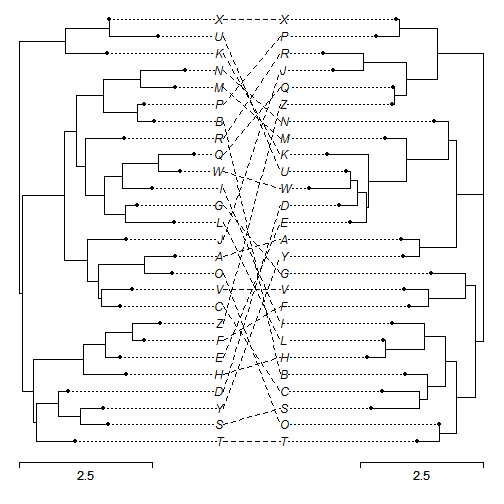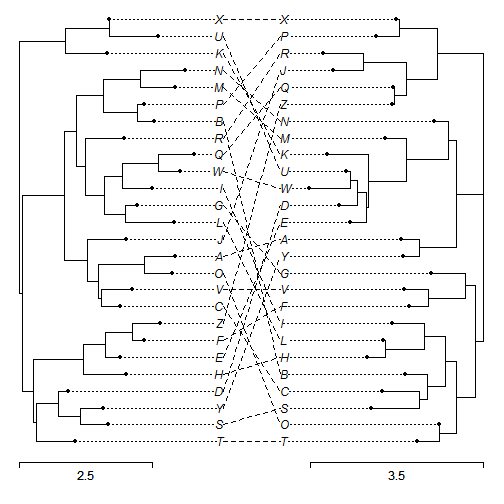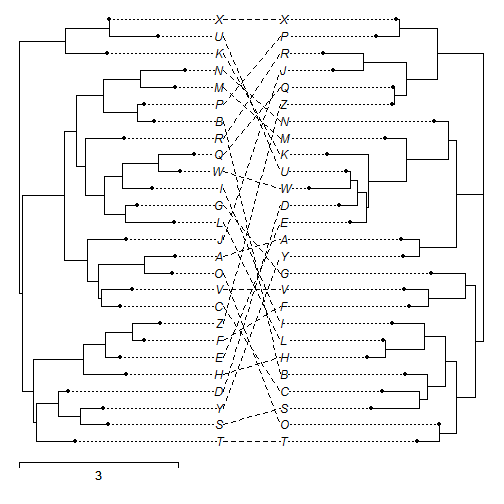## Saturday, October 10, 2015

### Scale bar for cophylo plotting

I just added the option to the phytools function `cophylo` (for co-phylogenetic plotting) to show separate scale bars for the right & left plotted trees.

Here's a quick demo of how it works:

``````library(devtools)
install_github("liamrevell/phytools",quiet=TRUE)
``````
``````## Installing 1 packages: expm
``````
``````## package 'expm' successfully unpacked and MD5 sums checked
``````
``````library(phytools)
``````
``````## Loading required package: ape
##
##  # ATTENTION: maps v3.0 has an updated 'world' map.        #
##  # Many country borders and names have changed since 1990. #
##  # Type '?world' or 'news(package="maps")'. See README_v3. #
``````

First, simulate some trees:

``````t1<-rtree(n=26,tip.label=LETTERS)
t2<-rtree(n=26,tip.label=LETTERS)
``````

The basic plots, no scale bar, look as follows:

``````obj<-cophylo(t1,t2)
``````
``````## Rotating nodes to optimize matching...
## Done.
``````
``````plot(obj)
``````Now let's try with the same scale bar, 2.5 units in length, on either side:

``````plot(obj,scale.bar=rep(2.5,2))
``````We can use different scale bars:

``````plot(obj,scale.bar=c(2.5,3.5))
``````And, finally, if we set either to zero it turns off:

``````plot(obj,scale.bar=c(3,0))
``````Note that the latter plot might be misinterpreted as a single scale bar for both the right & left plotted trees - but this would be wrong (unless the two trees happen to have exactly the same total height) as the trees are always rescaled internally before plotting in this function!

That's it!

#### 1 comment:

1.Is there is a way mot to rescale trees? This might be useful to compare chronograms.

Note: due to the very large amount of spam, all comments are now automatically submitted for moderation.